# OFFSET Function

The OFFSET function returns the value in a cell which is an offset from another cell.
This function does not move any cells or change the selection.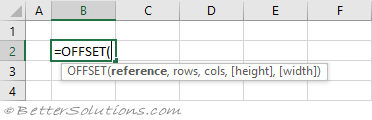### Return a Single Value (below)

You can return the value from a cell which is a certain number of rows below another cell.
The reference cell is "B2".
The row for the cell we want to return is 3 rows below the reference cell. This value is in cell "E2".
The column for the cell we want to return is 0 columns to the right of the reference cell. This value is 0.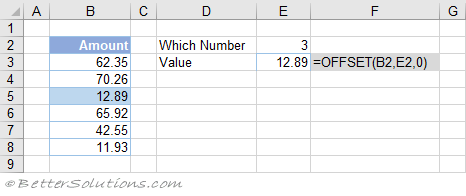### Return a Single Value (below and right)

You can return the value from a cell which is a certain number of rows below and a certain number of columns to the right of another cell.
The reference cell is "B2".
The row for the cell we want to return is 3 rows below the reference cell. This value is in cell "C8".
The column for the cell we want to return is 3 columns to the right of the reference cell. This value is in cell "C9".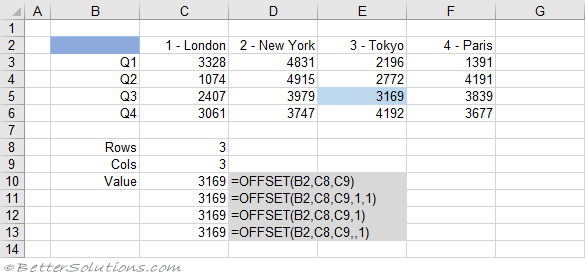### Return a Single Value (above and left)

You can return the value from a cell which is a certain number of rows above and a certain number of columns to the left of another cell.
The reference cell is "G7".
The row for the cell we want to return is 2 rows above the reference cell. This value is in cell "C8".
The column for the cell we want to return is 2 columns to the left of the reference cell. This value in in cell "C9".
Notice that the numbers in cells "C8" and "C9" are negative.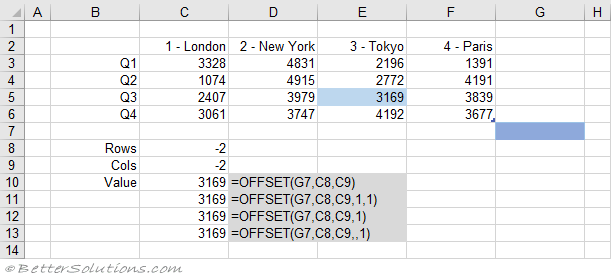### Return Multiple Values (positive height)

You can return multiple values from cells which are a certain number of rows below and a certain number of columns to the right of another cell.
The reference cell is "B2".
The first row for the cells we want to return is 2 rows below the reference cell. This value is in cell "C8".
The first column for the cells we want to return is 3 columns to the right of the reference cell. This value in in cell "C9".
The size of the range we want to return is 1 cell high. This height value is in cell "C10".
The size of the range we want to return is 2 cells wide. This width value is in cell "C11".
This must be entered as an Array Formula using (Ctrl + Shift + Enter).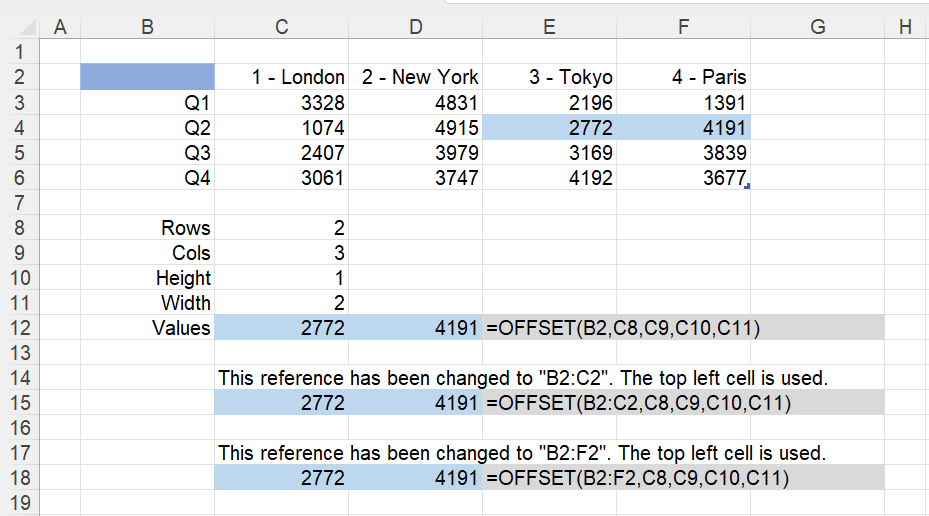### Return Multiple Values (reference range)

Using a single reference cell and explicitly providing the height and width arguments is best practice.
However you can achieve the same result by not providing a height and a width but increasing the size of the "reference".
When the height and width are not provided, the returned range is assumed to be the same size as the "reference".
When you enter a "reference" that contains more than one cell the top left cell is used for the offset.

The reference range is "B2:C2".
The first row for the cells we want to return is 2 rows below the reference cell. This value is in cell "C8".
The first column for the cells we want to return is 3 columns to the right of the reference cell. This value in in cell "C9".
The height is not provided but is assumed to be 1, which is the height of the "reference".
The width is not provided but is assumed to be 2, which is the width of the "reference".
The returned range is then expanded to include a second column because width is 2.
This must be entered as an Array Formula using (Ctrl + Shift + Enter).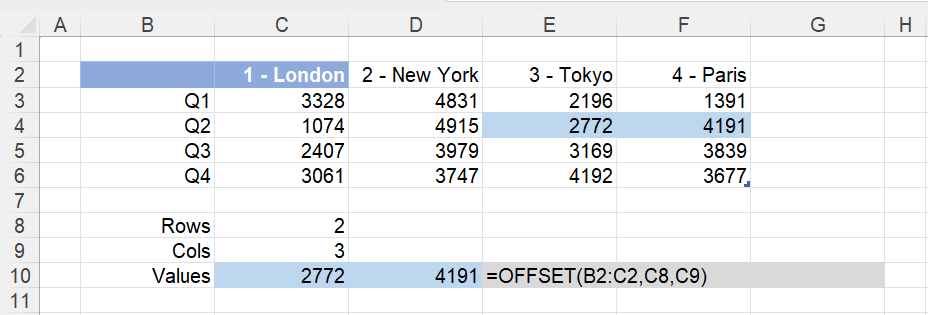### Return Multiple Values (negative height)

You can return the values from a range which is a certain number of rows and columns below a specific range.
This will offset 3 rows "down" (positive number) and 4 columns "to the right" (positive number), to cell "F5".
The range will then be expanded "up" (negative number) to include 2 rows in total.
The range will then be expanded "to the left" (negative number) to include 4 columns in total.
The reference cell is "B2".
This must be entered as an Array Formula using (Ctrl + Shift + Enter).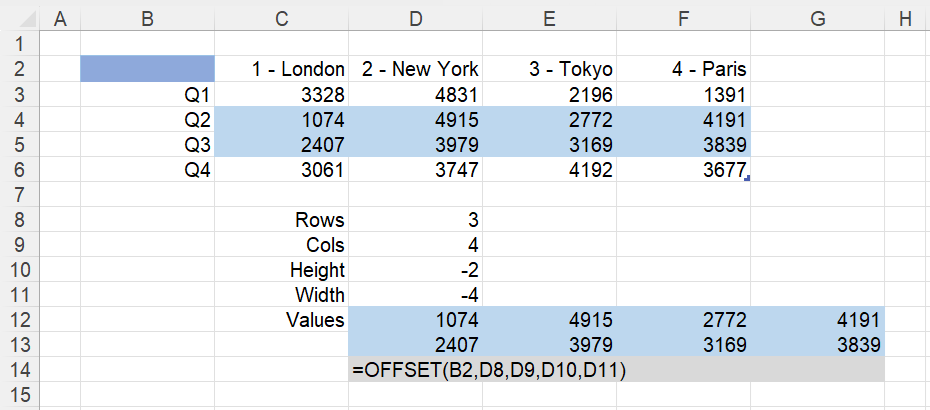### Return Multiple Values (negative rows and cols)

This will offset 3 rows "up" (negative number) and 4 columns "to the left" (negative number), to cell "C4".
The range will then be expanded "down" (positive number) to include 2 rows in total.
The range will then be expanded to the "right" (positive number) to include 4 columns in total.
The reference cell is "G7".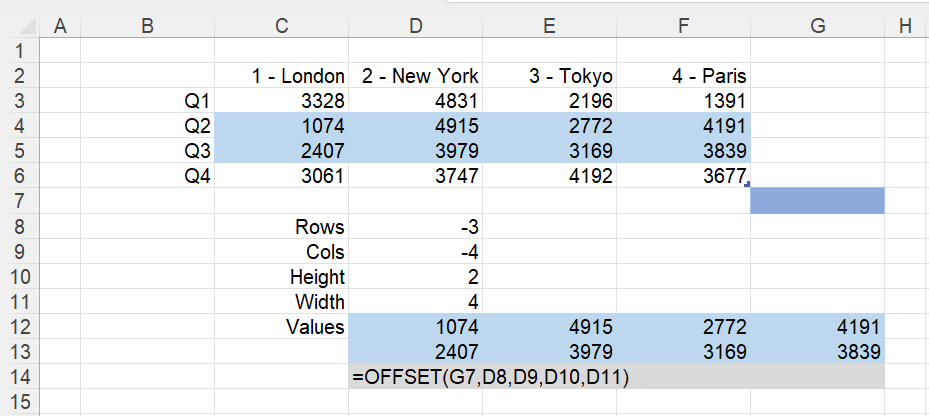### Return Multiple Values (nested)

You can use this function to pass multiple values into other functions.
The reference cell is "B2".
The first row for the cells we want to return is 3 rows below the reference cell.
The first column for the cells we want to return is 3 columns to the right of the reference cell.
The size of the range we want to return is 2 cells high.
The size of the range we want to return is 2 cells wide.
The returned range is then expanded to include a second column because width is 2.
This range of cells is then passed to the SUM function.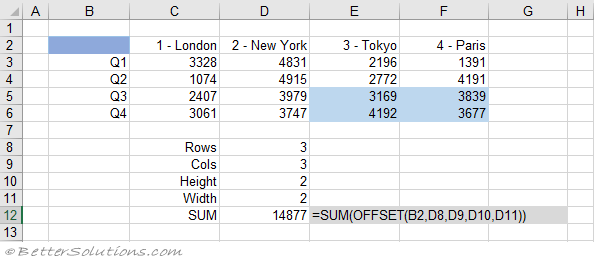### Related Formulas

Average the last n numbers
Sum the bottom n smallest numbers
Dynamic sum formula =SUM(B2.OFFSET(B10,-1,0)
Dynamic named ranges